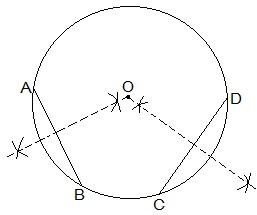Guru

# Suppose you are given a circle. Give a construction to find its centre. Q.2

• 0

What is the best way for solving the question from class 9th ncert math of Circles chapter of Ncert of exercise 10.3 of math. What is the best way for solving this question please guide me the best way for solving this question no.2 Suppose you are given a circle. Give a construction to find its centre.

Share

1.The construction steps to find the center of the circle are:

Step I: Draw a circle first.

Step II: Draw 2 chords AB and CD in the circle.

Step III: Draw the perpendicular bisectors of AB and CD.

Step IV: Connect the two perpendicular bisectors at a point. This intersection point of the two perpendicular bisectors is the centre of the circle.

• 0